# MCAT General Chemistry Review Summary

## Gold Standard MCAT General Chemistry Review

This MCAT General Chemistry Review Summary Page is by no means an exhaustive review of MCAT General Chemistry. Our summary is only meant to highlight key points that are most helpful for the MCAT. For a list of topics for the MCAT: MCAT General Chemistry Topics List. You can also access practice questions in our Free MCAT practice test , or our many full-length MCAT practice tests.

For MCAT General Chemistry, it helps to understand vocabulary, definitions and equations. In some respects, this General Chemistry cheat sheet will minimize your need to memorize information and maximize your General Chemistry review.

## Gold Standard MCAT General Chemistry Review: Stoichiometry

Note: Unless mentioned otherwise, the following images are excerpts from the Gold Standard MCAT General Chemistry ebook.

• Mole - Atomic and Molecular Weights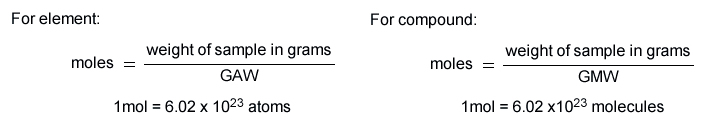• Categories of Chemical Reactions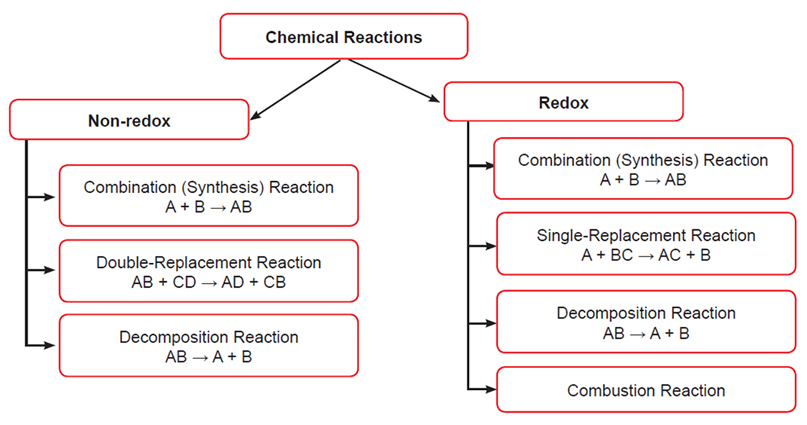Note: Any reaction that does not involve the transfer of electrons (= change in oxidation numbers) qualifies as a non-redox reaction. Combination reactions qualify as non-redox reactions when all reactants and products are compounds and the oxidation numbers do not change. Decomposition reactions qualify as non-redox reactions when all reactants and products are compounds and the oxidation numbers do not change. Additional information: UNC-Chapel Hill Chemistry Fundamentals Program

• Oxidation Numbers, Redox Reactions, Oxidizing vs. Reducing Agents

• Here are the general rules:
• In elementary substances, the oxidation number of an uncombined element is zero
• In monatomic ions the oxidation number of the elements that make up this ion is equal to the charge of the ion
• In a neutral molecule the sum of the oxidation numbers of all the elements that make up the molecule is zero
• Some useful oxidation numbers to memorize
• For H: +1, except in metal hydrides where it is equal to -1
• For O: -2 in most compounds; In peroxides (e.g. in H2O2) the oxidation number for O is -1, it is +2 in OF2 and -1/2 in superoxides
• For alkali metals: +1
• For alkaline earth metals: +2
• Aluminium always has an oxidation number of +3 in all its compounds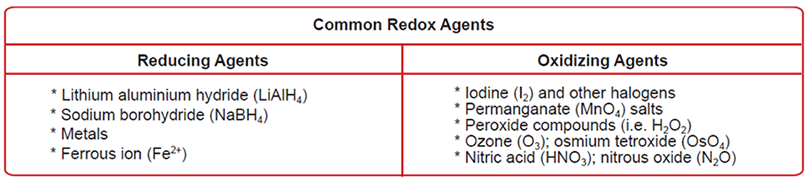• Mixtures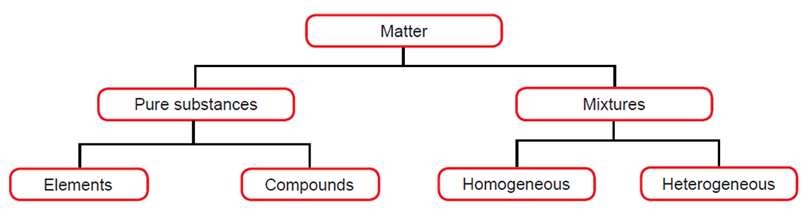## Gold Standard MCAT General Chemistry Review: Electronic Structure & The Periodic Table

• Conventional Notation for Electronic Structure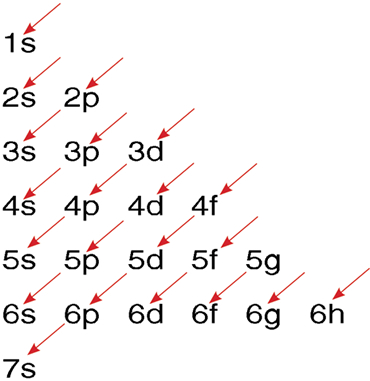The order for filling atomic orbitals: Follow the direction of successive arrows moving from top to bottom.

• Metals, Nonmetals and Metalloids

*General Characteristics of metals, nonmetals and metalloids

Metals Nonmetals Metalloids
Hard and shiny Gases or dull, brittle solids Appearance will vary
3 or less valence electrons 5 or more valence electrons 3 to 7 valence electrons
Form + ions by losing e Form － ions by gaining e Form + and/or －ions
Good conductors of heat and electricity Poor conductors of heat and electricity Conduct better than nonmetals, but not as well as metals
*These are general characteristics. There are exceptions beyond the scope of the exam.

## Gold Standard MCAT General Chemistry Review: Bonding

• Partial Ionic Character
• This polar bond will also have a dipole moment given by:where q is the charge and d is the distance between these two atoms.
• Lewis Acids and Lewis Bases
• The Lewis acid BF3 and the Lewis base NH3. Notice that the green arrows follow the flow of electron pairs.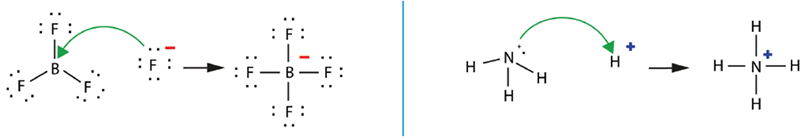• Valence Shell Electronic Pair Repulsions (VSEPR Models)
• Geometry of simple molecules in which the central atom A has one or more lone pairs of electrons (= e-)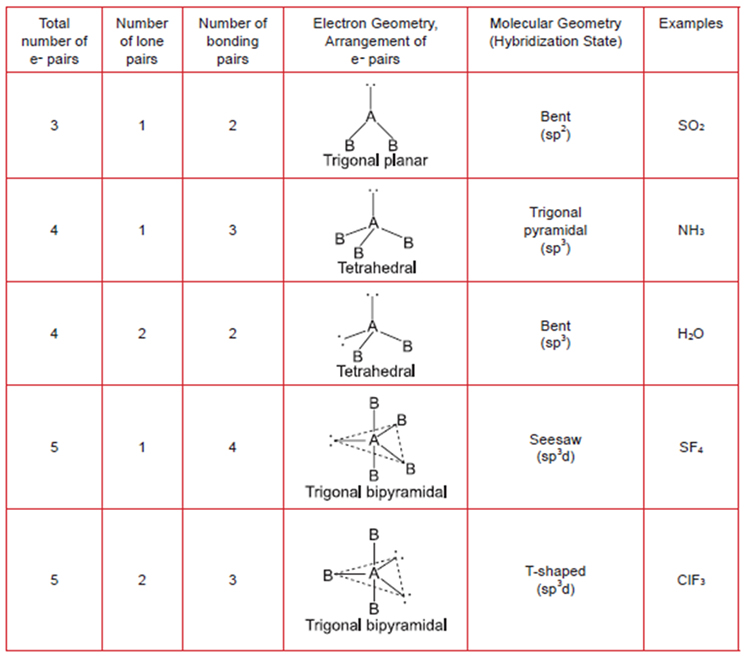Note: dotted lines only represent the overall molecular shape and not molecular bonds. In brackets under "Molecular Geometry" is the hybridization as discussed in ORG 1.2.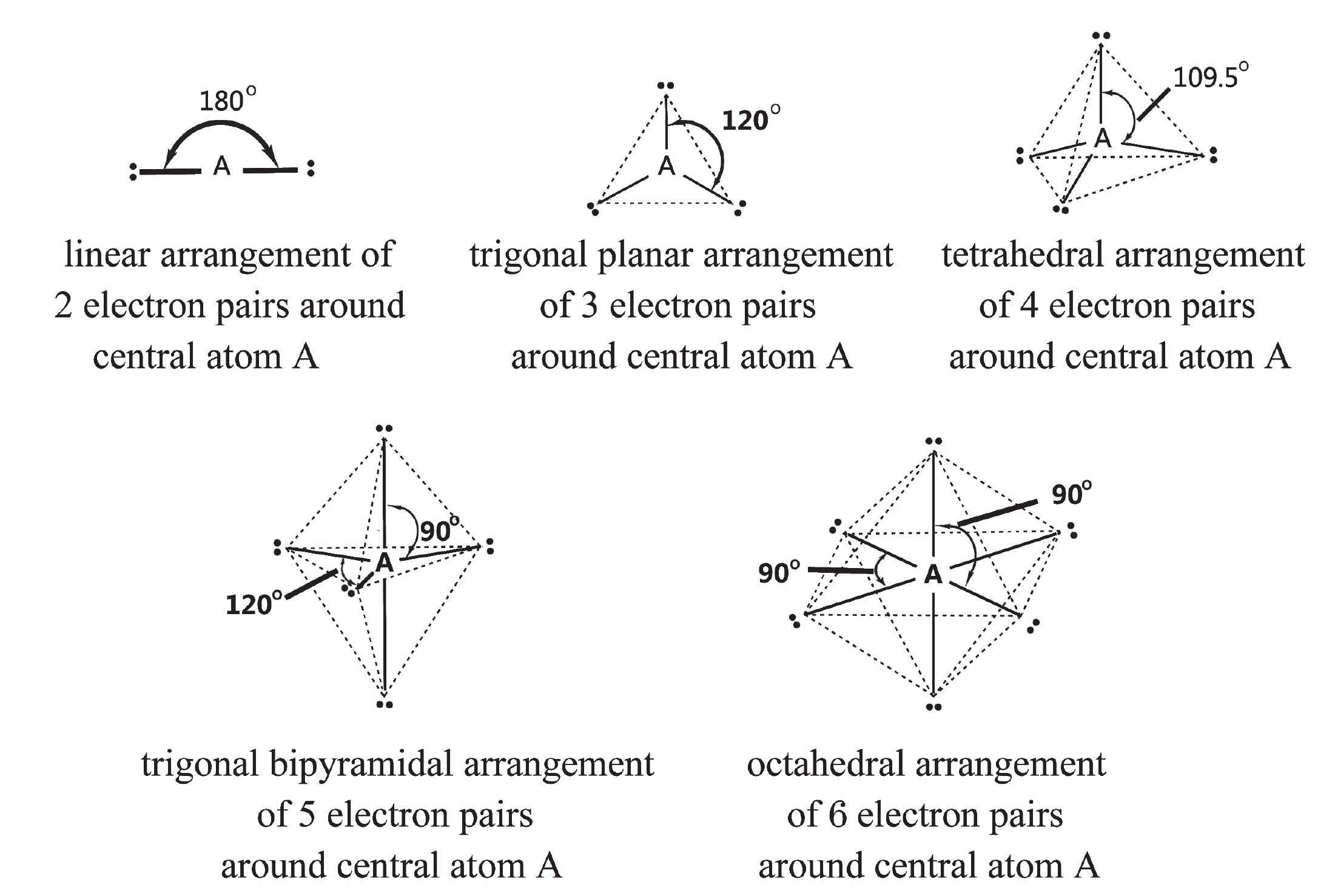Molecular arrangement of electron pairs around a central atom A. Dotted lines only represent the overall molecular shape and not molecular bonds.

## Gold Standard MCAT General Chemistry Review: Phases & Phase Equilibria

• Standard Temperature and Pressure, Standard Molar Volume
• 0 °C (273.15 K) and 1.00 atm (101.33 kPa = 760 mmHg = 760 torr); these conditions are known as the standard temperature and pressure (STP). {Note: the SI unit of pressure is the pascal (Pa).}
• The volume occupied by one mole of any gas at STP is referred to as the standard molar volume and is equal to 22.4 L.
• Kinetic Molecular Theory of Gases (A Model for Gases)
• The average kinetic energy of the particles (KE = 1/2 mv2) increases in direct proportion to the temperature of the gas (KE = 3/2 kT) when the temperature is measured on an absolute scale (i.e. the Kelvin scale) and k is a constant (the Boltzmann constant).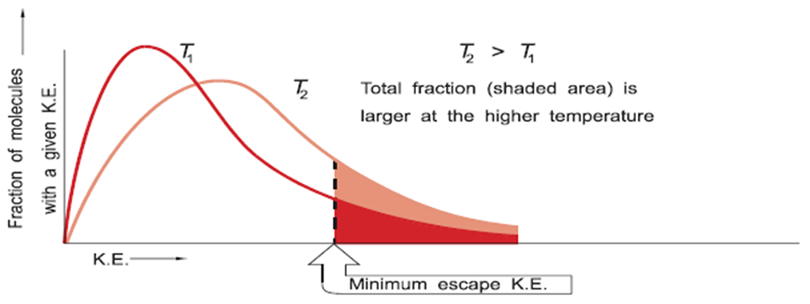The Maxwell Distribution Plot

 Graham’s Law (Diffusion and Effusion of Gases)Combined Gas Law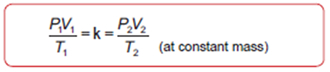Charles’ Law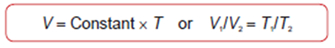Ideal Gas Law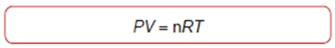since m/V is the density (d) of the gas: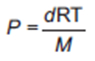Boyle’s Law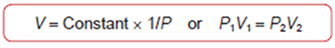Partial Pressure and Dalton’s Law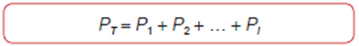Of course, the sum of all mole fractions in a mixture must equal one: ΣX1 = 1 Avogadro’s Law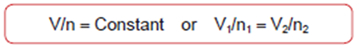The partial pressure (Pi) of a component of a gas mixture is equal to: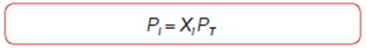• Liquid Phase (Intra- and Intermolecular Forces)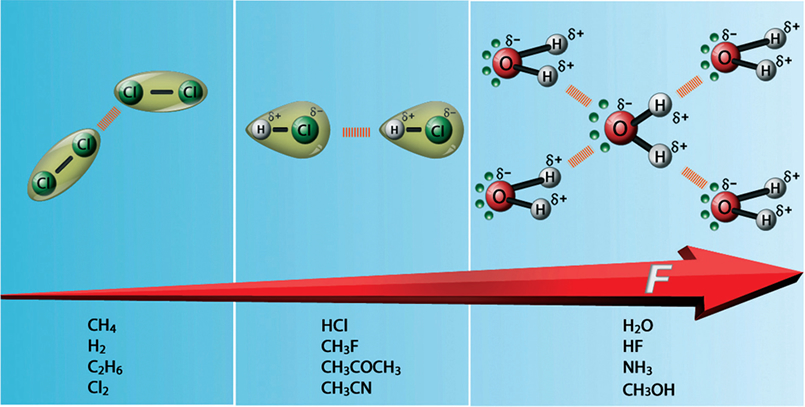Van Der Waal's forces (weak) and hydrogen bonding (strong). London forces between Cl2 molecules, dipole-dipole forces between HCl molecules and H-bonding between H2O molecules. Note that a partial negative charge on an atom is indicated by ẟ- (delta negative), while a partial positive charge is indicated by ẟ+ (delta positive). Notice that one H2O molecule can potentially form 4 H-bonds with surrounding molecules which is highly efficient. The preceding is one key reason that the boiling point of water is higher than that of ammonia, hydrogen fluoride, or methanol.

• Surface Tension
• PE is directly proportional to the surface area (A)
• PE = gA; g = surface tension
• g = F/l; F = force of contraction of surface; l = length along surface
•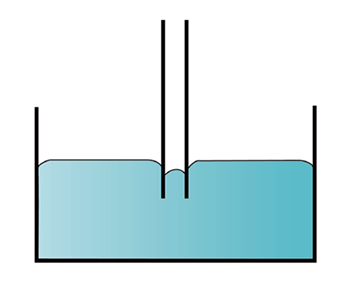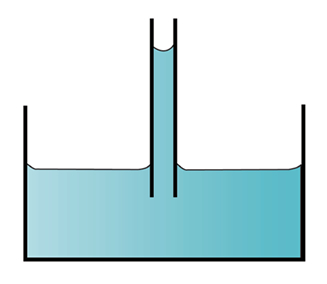• Phase Changes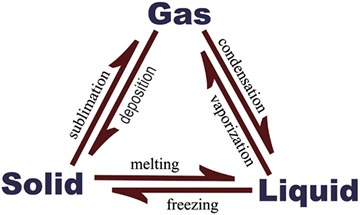• Phase Diagrams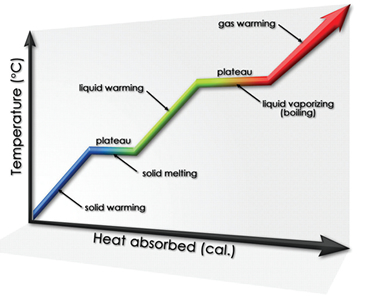## Gold Standard MCAT General Chemistry Review: Solution Chemistry

• Vapor-Pressure Lowering (Raoult’s Law)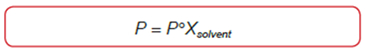where P = vapor pressure of solution
P° = vapor pressure of pure solvent (at the same temperature as P)
• Osmotic Pressure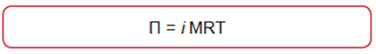where R = gas constant per mole
T = temperature in degrees K and
M = concentration of solute (mole/liter)
i = Van’t Hoff factor
• Boiling-Point Elevation and Freezing-Point Depression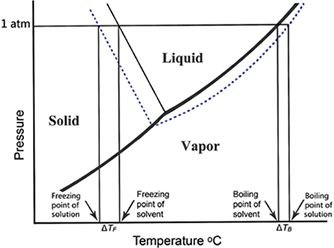Phase diagram of water demonstrating the effect of the addition of a solute

ΔTB=iKbm
ΔTF=iKFm
• Ions in Solution
• Ions that are positively charged = cations; ions that are negatively charged = anions
• Mnemonic: anions are negative ions
• The word “aqueous” simply means containing or dissolved in water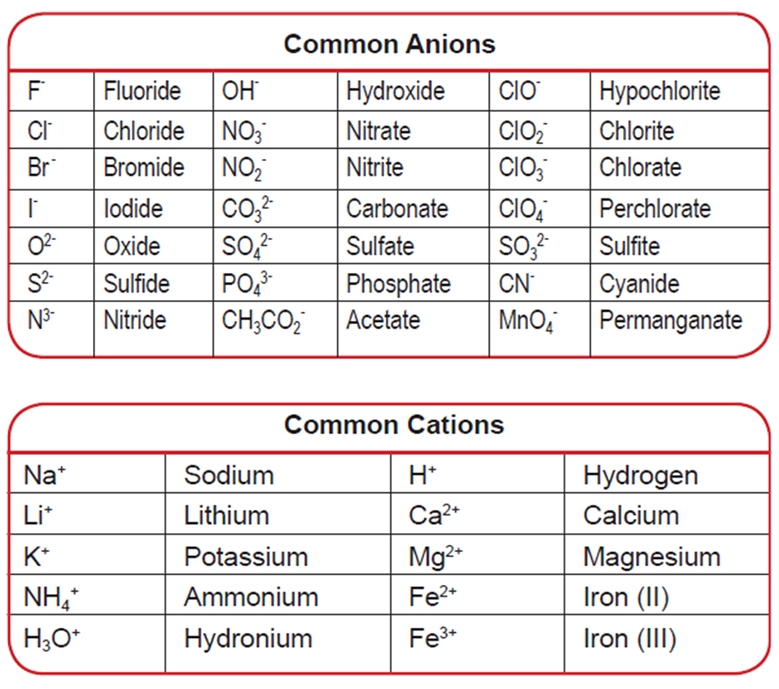Common Anions and Cations

• Units of Concentration
• Molarity (M): moles of solute/liter of solution (solution = solute + solvent)
• Normality (N): one equivalent per liter
• Molality (m): one mole/1000g of solvent
• Molal concentrations are not temperature-dependent as molar and normal concentrations are
• Density (ρ): Mass per unit volume at the specified temperature
• Osmole (Osm): The number of moles of particles (molecules or ions) that contribute to the osmotic pressure of a solution
• Osmolarity: osmoles/liter of solution
• Osmolality: osmoles/kilogram of solution
• Mole Fraction: amount of solute (in moles) divided by the total amount of solvent and solute (in moles)
• Dilution: MiVi = MfVf
• Solubility Product Constant, the Equilibrium Expression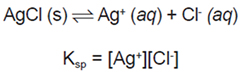Because the Ksp product always holds, precipitation will not take place unless the product of [Ag+] and [Cl-] exceeds the Ksp.

• Solubility Rules
1. All salts of alkali metals are soluble.
2. All salts of the ammonium ion are soluble.
3. All chlorides, bromides and iodides are water soluble, with the exception of Ag+, Pb2+, and Hg22+.
4. All salts of the sulfate ion (SO42-) are water soluble with the exception of Ca2+, Sr2+, Ba2+, and Pb2+.
5. All metal oxides are insoluble with the exception of the alkali metals and CaO, SrO and BaO.
6. All hydroxides are insoluble with the exception of the alkali metals and Ca2+, Sr2+, Ba2+.
7. All carbonates (CO32-), phosphates (PO43-), sulfides(S2-) and sulfites (SO32-) are insoluble, with the exception of the alkali metals and ammonium.

## Gold Standard MCAT General Chemistry Review: Acids & Bases

• Acids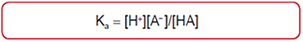STRONG WEAK
Perchloric HClO4
Chloric HClO3
Nitric HNO3
Hydrochloric HCl
Sulfuric H2SO4
Hydrobromic HBr
Hydriodic HI
Hydronium Ion H3O+
Hydrocyanic HCN
Hypochlorous HClO
Nitrous HNO2
Hydrofluoric HF
Sulfurous H2SO3
Hydrogen Sulfide H2S
Phosphoric H3PO4
Benzoic, Acetic and other Carboxylic Acids
• Water Dissociation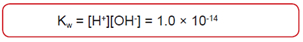• Salts of Weak Acids and Bases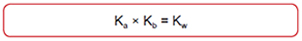• Buffers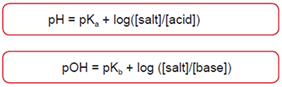• Bases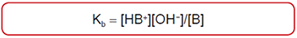• Strong bases include any hydroxide of the group 1A metals
• The most common weak bases are ammonia and any organic amine.
• Conjugate Acid-Base Pairs
• The acid, HA, and the base produced when it ionizes, A-, are called a conjugate acid-base pair.
• The pH Scaleat 25°C, pH + pOH = 14.0

• Properties of Logarithms
1. logaa = 1
2. logaMk = k logaM
3. loga(MN) = logaM + logaN
4. loga(M/N) = logaM - logaN
5. 10log10(M) = M

## Gold Standard MCAT General Chemistry Review: Thermodynamics

• The First Law of Thermodynamics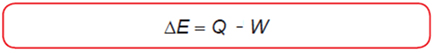• heat absorbed by the system: Q > 0
• heat released by the system: Q < 0
• work done by the system on its surroundings: W > 0
• work done by the surroundings on the system: W < 0
• Temperature Scales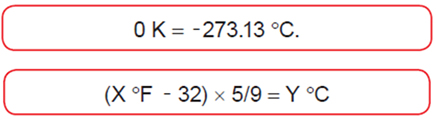• State Functions

• W can be determined experimentally by calculating the area under a pressure-volume curve

Work Heat Changes in internal energy
1st tranf. w 0 -w
2nd transf. W = w +q q -w

## Gold Standard MCAT General Chemistry Review: Enthalpy & Thermochemistry

• Heat of Reaction: Basic Principles
• A reaction during which heat is released is said to be exothermic (ΔH is negative).
• If a reaction requires the supply of a certain amount of heat it is endothermic (ΔH is positive).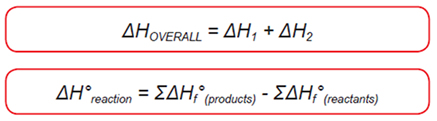• Bond Dissociation Energies and Heats of Formation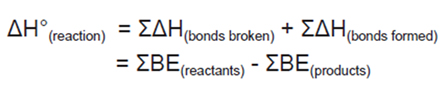• Calorimetry• The Second Law of Thermodynamics

• For any spontaneous process, the entropy of the universe increases which results in a greater dispersal or randomization of the energy (ΔS > 0).
• Entropy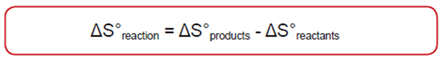• Free Energy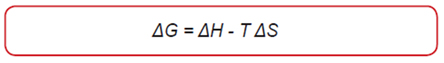• A reaction carried out at constant pressure is spontaneous if: ΔG < 0
• It is not spontaneous if: ΔG > 0
• It is in a state of equilibrium (reaction spontaneous in both directions) if: ΔG = 0

## Gold Standard MCAT General Chemistry Review: Rate Processes in Chemical Reactions

• Dependence of Reaction Rates on Concentration of Reactants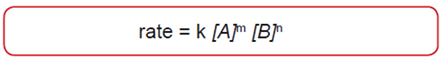• [ ] is the concentration of the corresponding reactant in moles per liter
• k is referred to as the rate constant
• m is the order of the reaction with respect to A
• n is the order of the reaction with respect to B
• m+n is the overall reaction order
• Dependence of Reaction Rates upon Temperature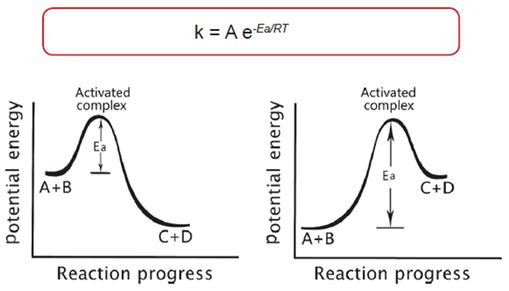Potential Energy Diagrams: Exothermic vs. Endothermic Reactions

• Catalysis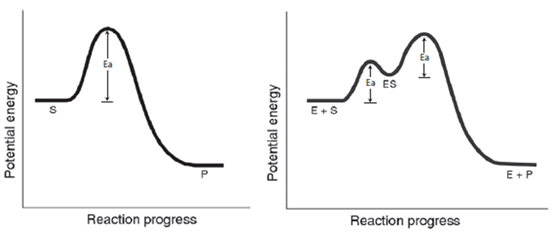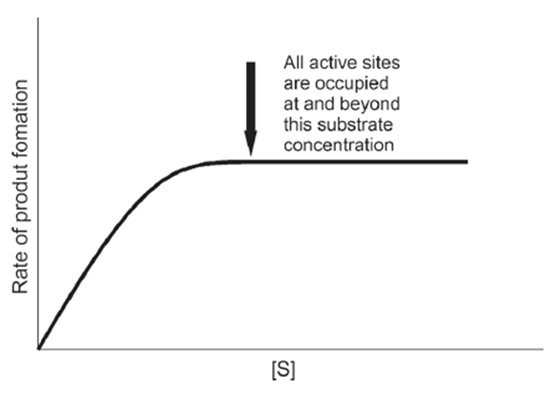Saturation Kinetics

• Equilibrium in Reversible Chemical Reactions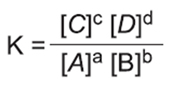• {Note: Catalysts speed up the rate of reaction without affecting Keq}

• Le Chatelier’s Principle

• Le Chatelier’s principle states that whenever a perturbation is applied to a system at equilibrium, the system evolves in such a way as to compensate for the applied perturbation.
• Relationship between the Equilibrium Constant and the Change in the Gibbs Free Energy

•• ## Gold Standard MCAT General Chemistry Review: Electrochemistry

• Generalities

• The more positive the E° value, the more likely the reaction will occur spontaneously as written.
• The strongest reducing agents have large negative E° values.
• The strongest oxidizing agents have large positive E° values.
• The oxidizing agent is reduced; the reducing agent is oxidized.
• Galvanic Cells

• Mnemonic: LEO is A GERC
• Lose Electrons Oxidation is Anode
• Gain Electrons Reduction at Cathode
• Concentration Cell

• Nernst equation
•• Faraday’s law relates the amount of elements deposited or gas liberated at an electrode due to current.
• One mole (= Avogadro’s number) of electrons is called a faraday (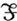).
• A faraday is equivalent to 96 500 coulombs.
• A coulomb is the amount of electricity that is transferred when a current of one ampere flows for one second (1C = 1A . S).

## Gold Standard MCAT General Chemistry Review: Laboratory

• Glassware

• Analyzing the Data

• The uncertain figure of a measurement is the last significant figure of the measurement.

### Examples of Significant Figures Versus Non-Significant Figures

Significant Examples Non-Significant
Non-zero integers 1.234 (4 sf)
Zeros between non-zero integers* 1.004 (4 sf)
Zeros to the right of decimal place AND integers* 1.2000 (5 sf)
0.0041 (2 sf)
Zeros to the right of decimal place BUT to the left of integers
430 (2 sf) Zeros to the left of decimal place BUT to the right of integers
013.2 (3 sf) Zeros to the left of decimal place AND integers

*Zeros in any number are used to convey either accuracy or magnitude. If a zero is to convey accuracy, then it is known as a significant value and if the zero is used to convey magnitude, then it is non-significant.